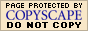Rational Expressions: Simplifying (page 2 of 3)

Sections: Finding the domain, Simplifying rational expressionsThinking back to when you were dealing with whole-number fractions, one of the first things you did was simplify them: You "cancelled off" factors which were in common between the numerator and denominator. You could do this because dividing any number by itself gives you just "1", and you can ignore factors of "1".

Using the same reasoning and methods, let's simplify some rational expressions.

• Simplify the following expression:
•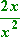To simplify a numerical fraction, I would cancel off any common numerical factors. For this rational expression (this polynomial fraction), I can similarly cancel off any common numerical or variable factors.

The numerator factors as (2)(x); the denominator factors as (x)(x). Anything divided by itself is just "1", so I can cross out any factors common to both the numerator and the denominator. Considering the factors in this particular fraction, I get: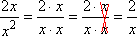Then the simplified form of the expression is: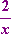• Simplify the following rational expression:
•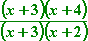How nice! This one is already factored for me! However (warning!), you will usually need to do the factorization yourself, so make sure you are comfortable with the process!

The only common factor here is "x + 3", so I'll cancel that off and get: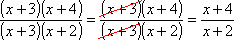Then the simplified form is: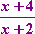Warning: The common temptation at this point is to try to continue on by cancelling off the 2 with the 4. But you cannot do this. Whenever you have terms added together, there are understood parentheses around them, like this: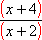You can only cancel off factors (that is, entire expressions contained within parentheses), not terms (that is, not just part of the contents of a pair of parentheses). To go inside the parentheses and try to cancel off part of the contents is like ripping off arms and legs of the poor little polynomial trapped inside. It'll be bleeding and oozing and flopping around on the floor, whimpering plaintively while sadly gazing up at you with big brown eyes...

Well, okay; maybe not. But trying to cancel off only a portion of a factor would be like trying to do this: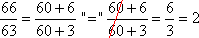Is 66/63 equal to 2? Of course not. And if the above "cancellation" is illegitimate, then so also is this one: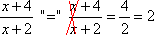...and it's illegitimate for EXACTLY THE SAME REASON as the previous one was. While it isn't quite so obvious that you're doing something wrong in the second case with the variables, these two "cancellations" are not allowed because you're reaching inside the factors (the 66 and 63 above, and the x + 4 and x + 2 here) and ripping off parts of them, rather than cancelling off an entire factor.

You can only cancel factors, not terms!

You can use the Mathway widget below to practice simplifying a rational expression. Try the entered exercise, or type in your own exercise. Then click the "paper-airplane" button to compare your answer to Mathway's. (Or skip the widget and continue with the lesson.)

(Clicking on "Tap to view steps" on the widget's answer screen will take you to the Mathway site for a paid upgrade.)

There is one technical consideration which is often overlooked in algebra, but crops up later in calculus. In the exercise above, when I went from the original expression: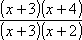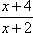...I removed a "division by zero" problem. That is, in the original fraction, I could not have plugged in the value x = −3, because this would have caused division by zero. But in the reduced fraction, x was allowed to be −3. But if the two expressions have different domains, can they really be equal? No, not exactly. Depended upon the text you're using, this technicality with the domain may be ignored or glossed over, or else you may be required to make note of it. Specifically, many (most?) textbooks will accept the following as your answer: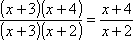...but some books (and instructors) will require that your simplified form be adjusted, as necessary, in order to have the same domain as the original form, so the technically-complete answer would be: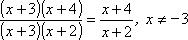Depending on your book and instructor, you may not need the "as long as x isn't equal to −3" part. If you're not sure which answer your instructor is expecting, ask now (before the test).

<< Previous  Top  |  1 | 2 | 3  |  Return to Index  Next >>

 Cite this article as: Stapel, Elizabeth. "Rational Expressions: Simplifying." Purplemath. Available from     https://www.purplemath.com/modules/rtnldefs2.htm. Accessed [Date] [Month] 2016

MathHelp.com Courses
This lesson may be printed out for your personal use.